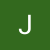# The Blind 75 Leetcode Series: Remove Nth Node From End of ListPhoto by Chris Ried on Unsplash

Today, we are working on Remove Nth Node From End of List.

Given the `head` of a linked list, remove the `nth` node from the end of the list and return its head.

Example 1:

Input: head = [1,2,3,4,5], n = 2
Output: [1,2,3,5]

Example 2:

Input: head = , n = 1
Output: []

Example 3:

Input: head = [1,2], n = 1
Output: 

Constraints:

The number of nodes in the list is `sz`.

`1 <= sz <= 30`

`0 <= Node.val <= 100`

`1 <= n <= sz`

Follow up: Could you do this in one pass?

The problem gives pretty clear instruction already. I think these constraints are well enough for us to work on, so let’s dive into it directly.

I was thinking what would be the most direct, brute-force approach. Normally, it would be a way to check “all the combinations”, but what would it be in this case?

Maybe instead we can check for all conditions and make sure the solution satisfies.

When a `n` is given, we can assume 3 things:

1. n-th node represents the beginning of the list (n=len(link_list))
2. n-th node represents the end of the list (n=1)
3. n-th node represents a node somewhere in the middle (1 < n < len(link_list))

Since we are checking n-th node from the end of the list, we can go through the entire list once, store all nodes, and check these 3 conditions accordingly.

`def removeNthFromEnd(head, n):    nodes = []    begin = ListNode()    begin.next = head    while head:        nodes.append(head)        head = head.next    # head, n = len(nodes)    if n == len(nodes):        begin.next = begin.next.next    # tail, n = 1    elif n == 1:        nodes[-2].next = None    # mid, len(nodes) > n > 1     else:        nodes[len(nodes) - n - 1].next = nodes[len(nodes) - n + 1]    return begin.next`

We start with initializing the the list to store all the nodes, a `begin` that points to the `head` as its `next` node, and then iterating through the entire linked list to get all the nodes and stored in the list.

Then we check for the 3 conditions.

First, if the n-th node from behind represents the beginning of the list, then we simply need to point our `begin.next` to the node after the head, which is `begin.next.next`. This is safe to do because even if `head.next` does not exist, `begin.next.next` will effectively point to the right null node (or `None`).

Second, if the n-th node from behind represents the end of the list, then we can eliminate the last node and point second the last node to a null node, thus, `nodes[-2].next = None`

Last, for any of the node in the middle, we just point the previous node’s `next` to the node after, effectively skipping the target node.

So what’s the time complexity? We only iterate through the list once and retrieving the element through index is constant, so we just have O(n)

`def removeNthFromEnd(head, n):    nodes = []    begin = ListNode()    begin.next = head    while head:  # O(n)        nodes.append(head)        head = head.next    if n == len(nodes):        begin.next = begin.next.next    elif n == 1:        nodes[-2].next = None    else:        nodes[len(nodes) - n - 1].next = nodes[len(nodes) - n + 1]  # O(1) for get item    return begin.next`

What can be another solution? What if we have 2 pointers, one is `n` steps ahead of the other? Then we move both nodes toward the end of the list. When the node ahead reaches the end, the node behind will be at exactly `n-1 steps from the end of the list` , then we just need to point that node’s `next` to the node’s `next.next`, skipping the next node, and we are done.

Let’s take a look of the example given at the beginning another time for some visual aid

To put this in code:

`def removeNthFromEnd(head, n):    ahead = behind = head    for _ in range(n):  # put ahead pointer to position        ahead = ahead.next    if not ahead:  # linked list shorter than n, simply return head.next        return head.next    while ahead.next:  # check if ahead has reached to the end, if not move both pointers forwards        ahead = ahead.next        behind = behind.next    behind.next = behind.next.next  # skip the target node as now the behind node reaches 1 step before the target node    return head`

What’s the time complexity with this approach? We still only loop through the linked list once, so it’s still O(n)

`def removeNthFromEnd(head, n):    ahead = behind = head    for _ in range(n):  # O(1), constant to "n"        ahead = ahead.next    if not ahead:  # O(1)        return head.next    while ahead.next:  # O(n)        ahead = ahead.next        behind = behind.next    behind.next = behind.next.next  # O(1)    return head`

This approach is definitely cleaner as we don’t have to check each possible scenario, but it’s not necessarily faster. On average, the first approach reaches ~ 30ms run time which the second approach reaches ~55ms run time. Keep in mind that when you are whiteboarding, the run time is usually not shown, which means interviewers will usually look for a cleaner solution if the time complexity for all answers are the same. If you insist that your solution runs faster, you need to explain to the interviewers why it runs faster. We usually speak about time complexity using the worst case scenario, so maybe you can prove why your solution hits the worst case way less often than the other, cleaner approach. If you don’t think you can convince the interviewers, I’d stick with the cleaner solution. Remember, you only have ~ 45 mins and you want to make things as concise as possible.

There you have it! Another problem down!